•LarryDpk

# 1 Why we need partitioning

Table Partitioning can resolve some performance issue caused by big tables, such as query latency. If the size of one table is reaching to the memory of the machine, it suggests that you should partition the table. There are 3 forms for table partitioning:

• Range Partitioning
• List Partitioning
• Hash Partitioning

We will cover all the 3 forms by examples.

# 2 There way to partition

To make our work easier, we started up the PostgreSQL with Docker. We used the latest version so it can support Hash Partitioning:

``````docker run -itd \
--name pkslow-postgres \
-e POSTGRES_DB=pkslow \
-e POSTGRES_USER=pkslow \
-p 5432:5432 \
postgres:13
``````

## 2.1 Range Partitioning

We created the big table named `pkslow_person_r`, we would partition the table by age, the SQL as below:

``````CREATE TABLE pkslow_person_r (
age int not null,
city varchar not null
) PARTITION BY RANGE (age);
``````

Next we created the tables to partition the big table:表：

``````create table pkslow_person_r1 partition of pkslow_person_r for values from (MINVALUE) to (10);
create table pkslow_person_r2 partition of pkslow_person_r for values from (11) to (20);
create table pkslow_person_r3 partition of pkslow_person_r for values from (21) to (30);
create table pkslow_person_r4 partition of pkslow_person_r for values from (31) to (MAXVALUE);
``````

We created 4 tables:

• pkslow_person_r1: age from 0 to 10;
• pkslow_person_r2: age from 11 to 20;
• pkslow_person_r3: age from 21 to 30;
• pkslow_person_r4: age older than 30.

Let insert some test data:

``````insert into pkslow_person_r(age, city) VALUES (1, 'GZ');
insert into pkslow_person_r(age, city) VALUES (2, 'SZ');
insert into pkslow_person_r(age, city) VALUES (21, 'SZ');
insert into pkslow_person_r(age, city) VALUES (13, 'BJ');
insert into pkslow_person_r(age, city) VALUES (43, 'SH');
insert into pkslow_person_r(age, city) VALUES (28, 'HK');
``````

As you can see, the table name in `insert sql` is still `pkslow_person_r`, we don't need to use the partitioning sub tablename.

So as for querying: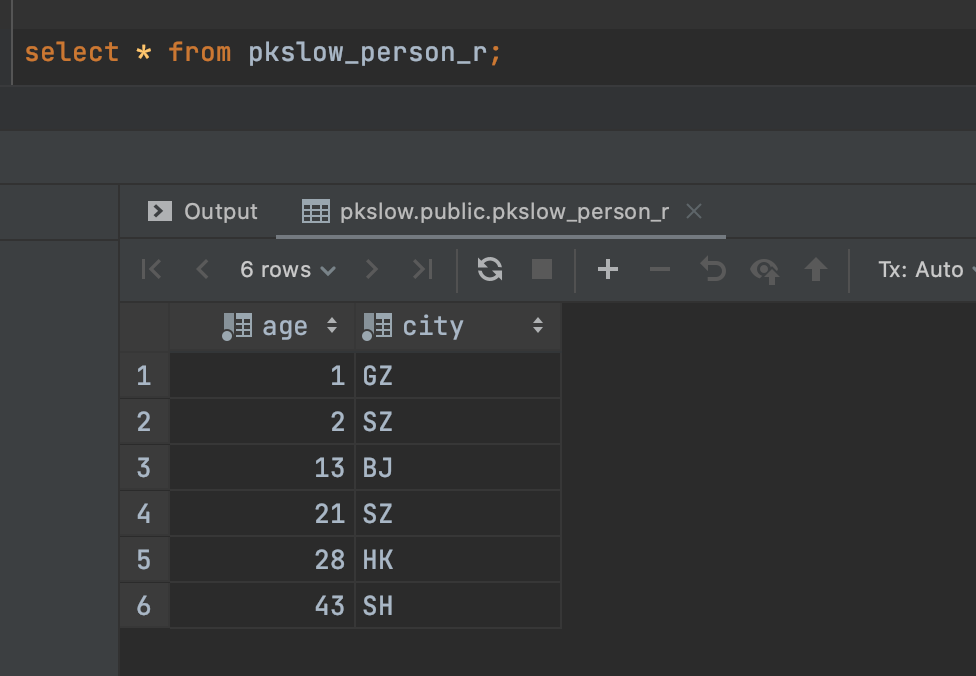But the 4 sub tables did exist: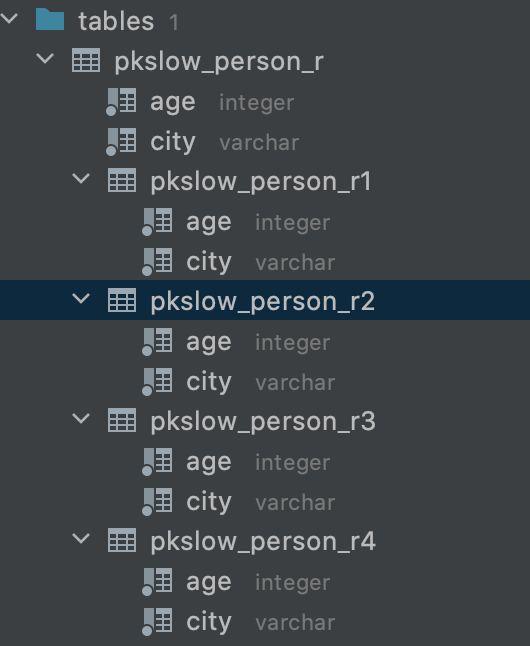If we queryed the sub table, we can just get the specific result belong to it: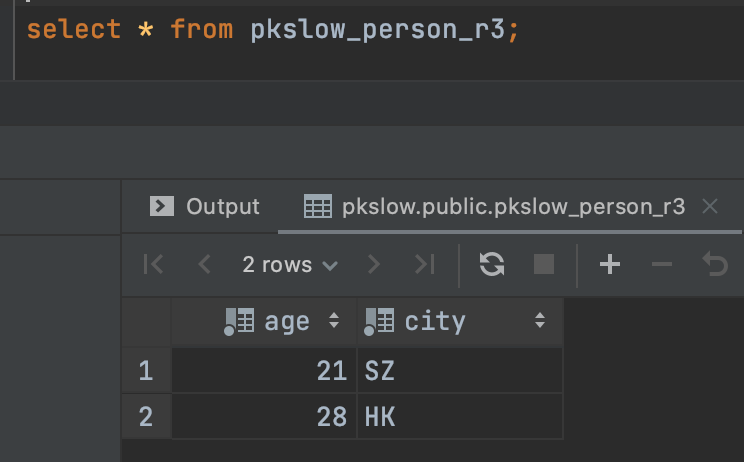## 2.2 List Partitioning

Similarly, List Partitioning can route your data into sub tables by the column name:

``````-- create the big table
create table pkslow_person_l (
age int not null,
city varchar not null
) partition by list (city);

-- create sub tables
CREATE TABLE pkslow_person_l1 PARTITION OF pkslow_person_l FOR VALUES IN ('GZ');
CREATE TABLE pkslow_person_l2 PARTITION OF pkslow_person_l FOR VALUES IN ('BJ');
CREATE TABLE pkslow_person_l3 PARTITION OF pkslow_person_l DEFAULT;

-- insert test data
insert into pkslow_person_l(age, city) VALUES (1, 'GZ');
insert into pkslow_person_l(age, city) VALUES (2, 'SZ');
insert into pkslow_person_l(age, city) VALUES (21, 'SZ');
insert into pkslow_person_l(age, city) VALUES (13, 'BJ');
insert into pkslow_person_l(age, city) VALUES (43, 'SH');
insert into pkslow_person_l(age, city) VALUES (28, 'HK');
insert into pkslow_person_l(age, city) VALUES (28, 'GZ');
``````

We queryed the first sub table, just data in city `GZ`: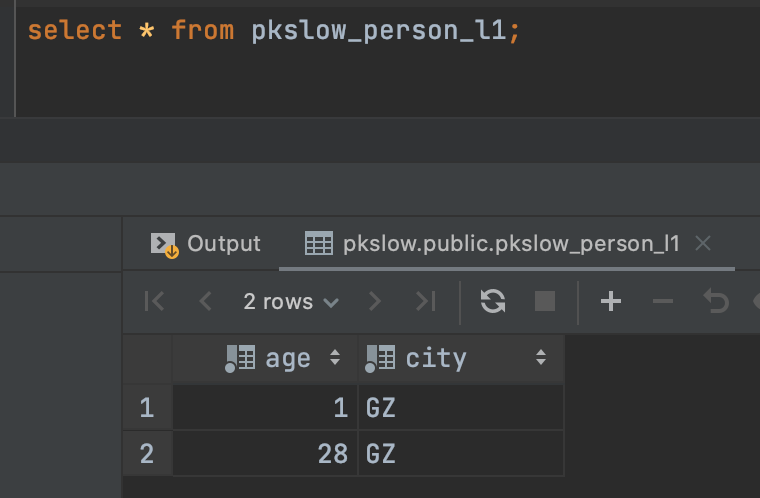## 2.3 Hash Partitioning

The Hash Partitioning can partition the big table by Hash value of column:

``````-- create the big table
create table pkslow_person_h (
age int not null,
city varchar not null
) partition by hash (city);

-- create the sub tables
create table pkslow_person_h1 partition of pkslow_person_h for values with (modulus 4, remainder 0);
create table pkslow_person_h2 partition of pkslow_person_h for values with (modulus 4, remainder 1);
create table pkslow_person_h3 partition of pkslow_person_h for values with (modulus 4, remainder 2);
create table pkslow_person_h4 partition of pkslow_person_h for values with (modulus 4, remainder 3);

-- insert the test data
insert into pkslow_person_h(age, city) VALUES (1, 'GZ');
insert into pkslow_person_h(age, city) VALUES (2, 'SZ');
insert into pkslow_person_h(age, city) VALUES (21, 'SZ');
insert into pkslow_person_h(age, city) VALUES (13, 'BJ');
insert into pkslow_person_h(age, city) VALUES (43, 'SH');
insert into pkslow_person_h(age, city) VALUES (28, 'HK');
``````

Queryed the one sub table: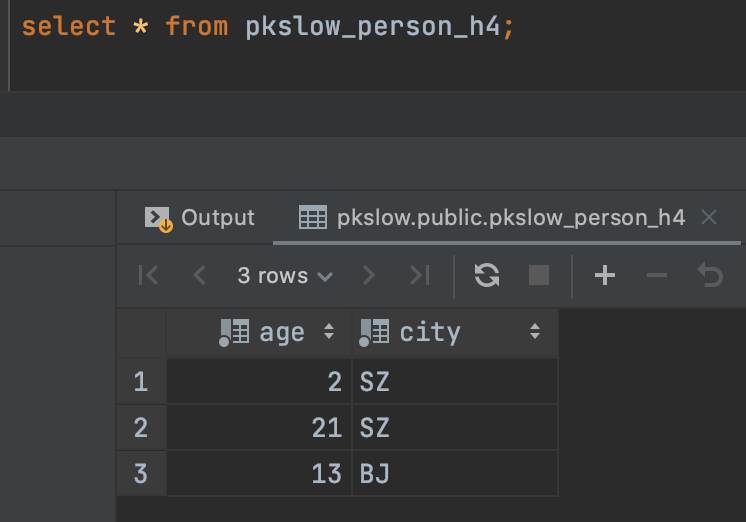# 3 sum-up

The code can check: https://github.com/LarryDpk/pkslow-samples

Reference:

Postgresql Table Partitioning

How to use table partitioning to scale PostgreSQL